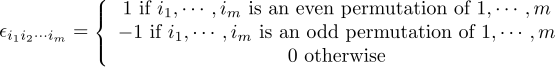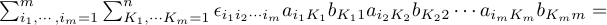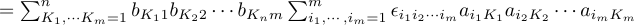Jump to content

# Determinants : Proof det(AB)=0 where Amxn and Bnxm with m>n

## Recommended Posts

Can someone help me?

I'm trying to proof this:

Proof det(AB)=0 where Amxn and Bnxm with m>n

I created a generic matrix A and B, then I use Laplace transforms to conclude.

I'd like to know if there is another way to proof that.

Thanks!

##### Share on other sites

Let A be a m×n matrix and B be a n×m matrix. If n<m then AB is not invertible.

Can someone proof this?
Could anybody help me?

##### Share on other sites

I don't know whether you're familiar with index notation. If you are, I think I can help you. If you aren't, I can't, because it's just too painful. They will have told you about Einstein's summation convention. Don't use it for this exercise, because if you do, you're as good as lost.

The key is: you need m indices that run from 1 to m, and another bunch of m indices that run from 1 to n

You also need the completely antisymmetric Lévi-Civita symbol:Now, the index that runs from 1 to n (the inner product index) I will call K1, ..., Kn

The other multi-index I will call i1,...im

And the third one, the second free index, I will fix to be 1, ..., m

Then,Now it takes a little insight: The last factor is the det of m vectors in an n-dimensional space. As m>n, it is therefore a linearly dependent set, so it must be zero. You can understand this better if you think of the det as a multilinear function of m vectors.

##### Share on other sites
15 hours ago, taeto said:

Can you use Gaussian elimination?

Gaussian elimination does not help here. The reason being that it requires you to reduce your matrix to a triangular form, and in order to do that, you need the actual expression of the matrix, not a generic amn

##### Share on other sites
45 minutes ago, joigus said:

Gaussian elimination does not help here.

It is perhaps not so clear.

We have a product $$AB$$ of matrices, where $$A$$ has more rows than columns.

Suppose that if we perform an elementary row operation on $$A$$ to produce a new matrix $$A',$$ then there is a matrix $$B'$$ for which $$AB=A'B'.$$ (This is supposed to be the only tricky part.)

Then since by elementary row operations we can bring $$A$$ into a matrix $$\bar{A}$$ that has at least one all-zero row, there will be a matrix $$\bar{B}$$ for which $$AB = \bar{A}\bar{B},$$ such that the latter matrix has at least one all-zero row.

Now it follows from the formula for the determinant that $$\bar{A}\bar{B},$$ and therefore $$AB,$$ has determinant zero.

##### Share on other sites
8 minutes ago, taeto said:

It is perhaps not so clear.

We have a product AB of matrices, where A has more rows than columns.

Suppose that if we perform an elementary row operation on A to produce a new matrix A, then there is a matrix B for which AB=AB. (This is supposed to be the only tricky part.)

Then since by elementary row operations we can bring A into a matrix A¯ that has at least one all-zero row, there will be a matrix B¯ for which AB=A¯B¯, such that the latter matrix has at least one all-zero row.

Now it follows from the formula for the determinant that A¯B¯,  and therefore AB, has determinant zero.

You may be right. Dimensional arguments could work. Let me think about it and get back to you in 6+ hours. I have a busy afternoon.

Thank you!

##### Share on other sites
8 hours ago, joigus said:

You may be right. Dimensional arguments could work. Let me think about it and get back to you in 6+ hours. I have a busy afternoon.

Thank you!

Yes, taeto, you are right, unless I'm too sleepy to think straight. The thing that's missing in your argument is the transformation matrix, which is, I think, what you mean by,

8 hours ago, taeto said:

This is supposed to be the only tricky part.

I don't know if you're aware of it, but any Gauss reduction operation can be implemented by a square non-singular matrix. A change-of-basis or "reshuffling" matrix. Let's call it D. So that,

AB = ADD-1B = A'B'

The "indexology" goes like this: (mxn)x(nxm) = (mxn)x(nxn)x(nxn)x(nxm)

The first factor would be an upper-triangular matrix (guaranteed by theorem that I can barely recall) but, as it has fewer columns than rows, at least the lower row must be the zero row, so that the product must have a zero row. Right?

(AAMOF you can do the same trick either by rows on the left or columns on the right; it's one or the other. Then you would have to apply a similar reasoning to B instead of A, you're welcome to fill in the details.)

This is like cracking nuts with my teeth to me, sorry. That's what I meant when I said,

23 hours ago, joigus said:

I don't know whether you're familiar with index notation. If you are, I think I can help you. If you aren't, I can't, because it's just too painful.

But that was a very nice piece of reasoning.

1 minute ago, joigus said:

A change-of-basis or "reshuffling" matrix.

It's actually not a change-of-basis matrix, but a completely different animal.

## Create an account or sign in to comment

You need to be a member in order to leave a comment

## Create an account

Sign up for a new account in our community. It's easy!

Register a new account

## Sign in

Already have an account? Sign in here.

Sign In Now
×

• #### Activity

• Leaderboard
×
• Create New...

## Important Information

We have placed cookies on your device to help make this website better. You can adjust your cookie settings, otherwise we'll assume you're okay to continue.